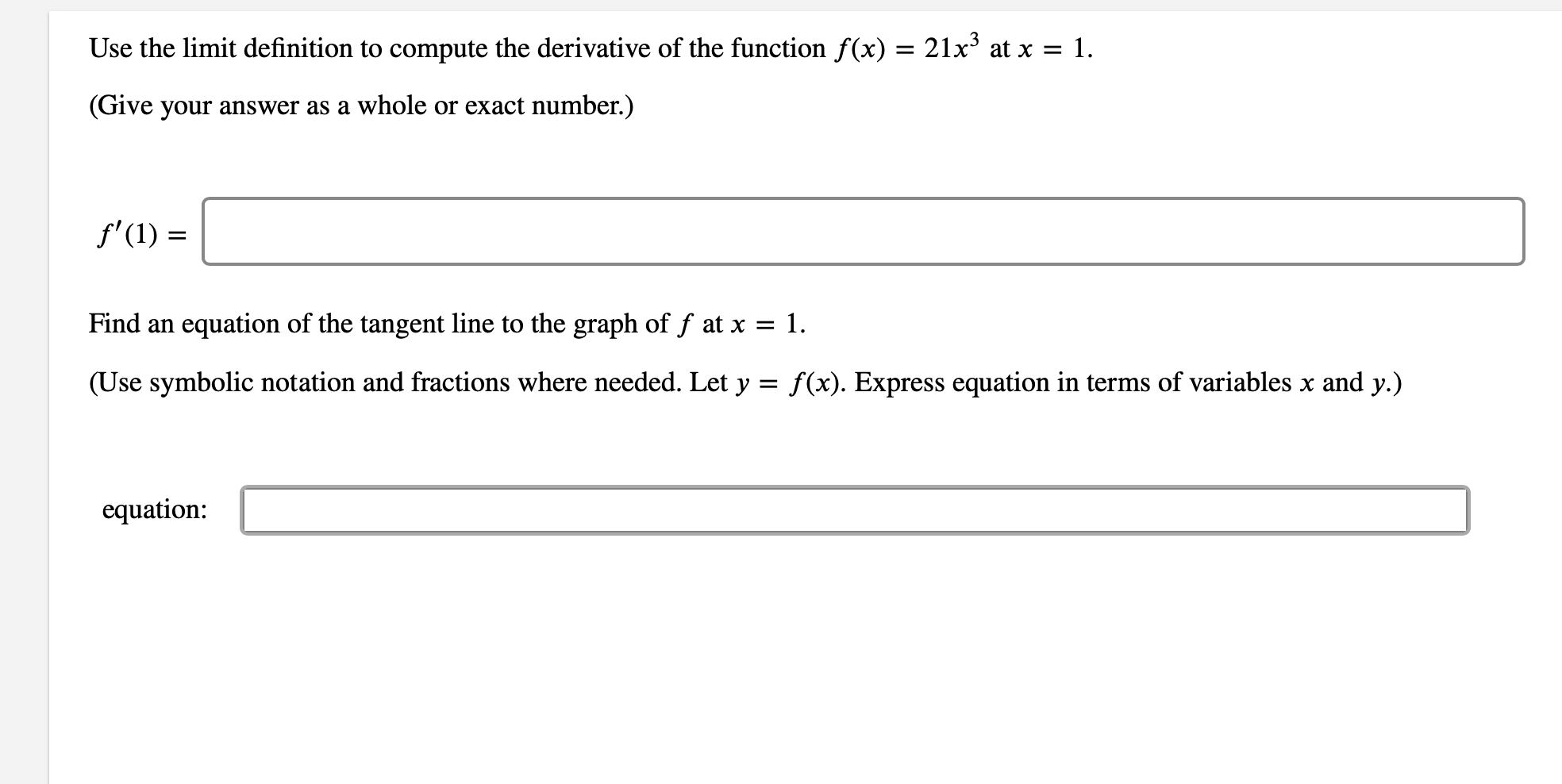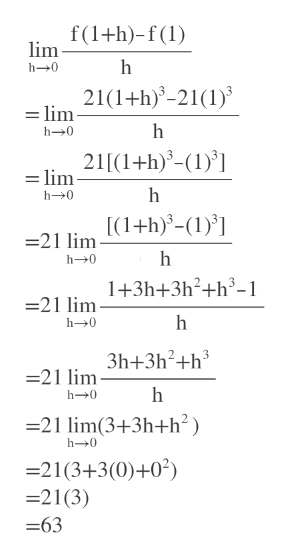# Use the limit definition to compute the derivative of the function f(x) = 21x3 at x = 1.(Give your answer as a whole or exact number.)f'(1)Find anequation of the tangent line to the graph of f at x = 1.(Use symbolic notation and fractions where needed. Let y =f(x). Express equation in terms of variables x and y.)equation

Question
3 viewshelp_outlineImage TranscriptioncloseUse the limit definition to compute the derivative of the function f(x) = 21x3 at x = 1. (Give your answer as a whole or exact number.) f'(1) Find an equation of the tangent line to the graph of f at x = 1. (Use symbolic notation and fractions where needed. Let y = f(x). Express equation in terms of variables x and y.) equation fullscreen
check_circle

Step 1

We use the definition of limit to find the derivative.help_outlineImage Transcriptionclosef(1+h)-f() lim h 0 21(1+h)3-21(1 = lim h 0 21[(1+h)-(1' = lim h h0 [(1+h)-(1) =21 lim h-0 1+3h+3h2+h3-1 21 lim h- 0 3h+3h2+h3 =21 lim h h- 0 -21 lim (3+3h+h2) h-0 21(3+3(0)+02) 21(3) =63 fullscreen
Step 2

At x=1 we have to find...

### Want to see the full answer?

See Solution

#### Want to see this answer and more?

Solutions are written by subject experts who are available 24/7. Questions are typically answered within 1 hour.*

See Solution
*Response times may vary by subject and question.
Tagged in

### Other